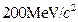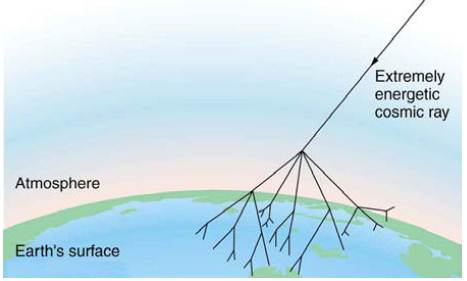Chapter 33, Problem 43PE

Chapter
Section
Textbook Problem

Integrated ConceptsThe intensity of cosmic ray radiation decreases rapidly with increasing energy, but there are occasionally extremely energetic cosmic rays that create a shower of radiation from all the particles they create by striking a nucleus in the atmosphere as seen in the figure given below. Suppose a cosmic ray particle having an energy ofconverts its energy into particles with masses averaging(a) How many particles are created? (b) If the particles rain down an a 1.00−km2 area, how many particles are there per square meter?Figure 33.27 An extremely energetic cosmic ray creates a shower of particles on earth. The energy of these rare cosmic rays can approach a joule (aboutand, after multiple collisions, huge numbers of panicles are created from this energy. Cosmic ray showers have been observed to extend over many square kilometers.

To determine

(a)

The number of particles created when cosmic ray particle having an energy of 1010 GeV

converts its energy into particles with masses averaging 200 MeV/c2.

Explanation

Given:

The incident cosmic ray particle having an energy of 1010 GeVconverts its energy into

particles with masses averaging 200 MeV/c2 is 5×1010.

Formula used:

Number of particles created

=Incident energy of cosmic ray/energy of single particle created

Calculation:

Number of particles created =1010GeV200MeV=

To determine

(b)

Number of particles per square meter if the particles rain down on a 1 km2 area.

Still sussing out bartleby?

Check out a sample textbook solution.

See a sample solution

The Solution to Your Study Problems

Bartleby provides explanations to thousands of textbook problems written by our experts, many with advanced degrees!

Get Started

What is a redox reaction?

Biology: The Unity and Diversity of Life (MindTap Course List)

Complete the following table:

Chemistry & Chemical Reactivity

In which domain and kingdom are humans classified?

Biology: The Dynamic Science (MindTap Course List)